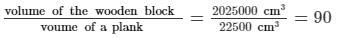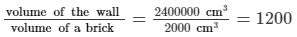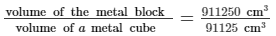# RD Sharma Solutions - Chapter 21 - Mensuration - II (Part - 2), Class 8, Maths Notes | Study RD Sharma Solutions for Class 8 Mathematics - Class 8

## Class 8: RD Sharma Solutions - Chapter 21 - Mensuration - II (Part - 2), Class 8, Maths Notes | Study RD Sharma Solutions for Class 8 Mathematics - Class 8

The document RD Sharma Solutions - Chapter 21 - Mensuration - II (Part - 2), Class 8, Maths Notes | Study RD Sharma Solutions for Class 8 Mathematics - Class 8 is a part of the Class 8 Course RD Sharma Solutions for Class 8 Mathematics.
All you need of Class 8 at this link: Class 8

PAGE NO 21.15:

Question 1:

Find the volume in cubic metre (cu. m) of each of the cuboids whose dimensions are:
(i) length = 12 m, breadth = 10 m, height = 4.5 cm
(ii) length = 4 m, breadth = 2.5 m, height = 50 cm.
(iii) length = 10 m, breadth = 25 dm, height = 50 cm.

(i)Length = 12 m

Height = 4.5 m

∴ Volume of the cuboid = length × breadth × height = 12 × 10 × 4.5 = 540 m³

(ii)Length = 4 m

Height = 50 cm

= 50/100m   (∵ 1 m  =  100 cm )

= 0.5 m

∴ Volume of the cuboid = length × breadth × height = 4 × 2.5 × 0.5 = 5 m³

(iii)Length = 10 m

= 25/10m (∵ 10 dm =  1m)

= 2.5 m

Height = 25 cm = 25/100m = 0.25 m

∴ Volume of the cuboid = length × breadth × height = 10 × 2.5 × 0.25 = 6.25 m³

Question 2:

Find the volume in cubic decimetre of each of the cubes whose side is
(i) 1.5 m
(ii) 75 cm
(iii) 2 dm 5 cm

(i)Side of the cube = 1.5 m

= 1.5 × 10 dm (∵ 1 m =  10 dm)

= 15 dm

∴ Volume of the cube = (side)3 = (15)3 = 3375 dm³

(ii)Side of the cube = 75 cm

= 75 × 1/10 dm (∵ 1 dm = 10 cm)

= 7.5 dm

∴ Volume of the cube = (side)3 = (7.5)3 = 421.875 dm³

(iii)Side of the cube  = 2 dm 5 cm

= 2 dm + 5 × 1/10 dm  (∵ 1 dm = 10 cm)

= 2 dm + 0.5 dm

= 2.5 dm

∴ Volume of the cube = (side)= (2.5)3 = 15.625 dm³

Question 3:

How much clay is dug out in digging a well measuring 3 m by 2 m by 5 m?

The measure of well is 3 m × 2 m × 5 m.

∴ Volume of the clay dug out = (3 × 2 × 5) m³ = 30 m³

Question 4:

What will be the height of a cuboid of volume 168 m³, if the area of its base is 28 m²?

Volume of the cuboid = 168 m³

Area of its base = 28 m²

Let h m be the height of the cuboid.

Now, we have the following:

Area of the rectangular base = length × breadth

Volume of the cuboid = length × breadth × height

⇒ Volume of the cuboid = (area of the base) × height

⇒ 168 = 28 × h

⇒ h = 168/28 = 6 m

∴ The height of the cuboid is 6 m.

Question 5:

A tank is 8 m long, 6 m broad and 2 m high. How much water can it contain?

Length of the tank = 8 m

Breadth = 6 m Height = 2 m

∴ Its volume = length × breadth × height = (8 × 6 × 2) m³ = 96 m³

We know that 1m³ = 1000 L

Now, 96 m³ = 96 × 1000 L = 96000 L

∴ The tank can store 96000 L of water.

:

Question 6:

The capacity of a certain cuboidal tank is 50000 liters of water. Find the breadth of the tank, if its height and length are 10 m and 2.5 m respectively.

Capacity of the cuboidal tank = 50000 L

1000 L = 1 m³

i.e., 50000 L = 50 × 1000 liters = 50 m³

∴ The volume of the tank is 50 m³.

Also, it is given that the length of the tank is 10 m.

Height  = 2.5 m

Suppose that the breadth of the tank is b m.

Now, volume of the cuboid = length × breadth × height

⇒ 50 = 10 × b × 2.5

⇒ 50 = 25 × b

⇒ b = 50/25 = 2 m

∴ The breadth of the tank is 2 m.

Question 7:

A rectangular diesel tanker is 2 m long, 2 m wide and 40 cm deep. How many liters of diesel can it hold?

Length of the rectangular diesel tanker = 2 m

Height = 40 cm

= 40 × 1/100 m   (∵ 1 m =  100 cm)

= 0.4 m

So, volume of the tanker = length × breadth × height = 2 × 2 × 0.4 = 1.6 m³

We know that 1 m³ = 1000 L

i.e., 1.6 m³ = 1.6 × 1000 L = 1600 L

∴ The tanker can hold 1600 L of diesel.

Question 8:

The length , breadth and height of a room are 5 m, 4.5 m and 3 m, respectively. Find the volume of the air it contains.

Length of the room = 5 m

Height = 3 m

Now, volume = length × breadth × height = 5 × 4.5 × 3 = 67.5 m³

∴ The volume of air in the room is 67.5 m³.

Question 9:

A water tank is 3 m long, 2 m broad and 1 m deep. How many litres of water can it hold?

Length of the water tank = 3 m

Breadth = 2 m Height = 1 m

Volume of the water tank = 3 × 2 × 1 = 6 m³

We know that 1 m³ = 1000 L

i.e., 6 m³ = 6 × 1000 L = 6000 L

∴ The water tank can hold 6000 L of water in it.

Question 10:

How many planks each of which is 3 m long, 15 cm broad and 5 cm thick can be prepared from a wooden block 6 m long, 75 cm broad and 45 cm thick?

Length of the wooden block = 6 m

= 6 × 100 cm   (∵ 1 m =  100 cm)

= 600 cm

Breadth of the block = 75 cm

Height of the block = 45 cm

Volume of block = length × breadth × height

= 600 × 75 × 45

= 2025000 cm³

Again, it is given that the length of a plank = 3 m

= 3 × 100 cm  (∵ 1 m =  100 cm)

= 300 cm

Breadth = 15 cm, Height = 5 cm

Volume of the plank = length × breadth × height

= 300 × 15 × 5 = 22500 cm³

∴ The number of such planks =Question 11:

How many bricks each of size 25 cm × 10 cm × 8 cm will be required to build a wall 5 m long, 3 m high and 16 cm thick, assuming that the volume of sand and cement used in the construction is negligible?

Dimension of a brick = 25 cm × 10 cm × 8 cm

Volume of a brick = 25 cm × 10 cm × 8 cm

= 2000 cm³

Also, it is given that the length of the wall is 5 m

= 5 × 100 cm  (∵1 m =  100 cm)

= 500 cm

Height of the wall = 3 m

= 3 × 100 cm   (∵ 1 m =  100 cm)

= 300 cm

It is 16 cm thick,

Volume of the wall = length × breadth × height = 500 × 300 × 16 = 2400000 cm³

∴ The number of bricks needed to build the wall =Question 12:

A village, having a population of 4000, requires 150 litres water per head per day. It has a tank which is 20 m long, 15 m broad and 6 m high. For how many days will the water of this tank last?

A village has population of 4000 and every person needs 150 L of water a day. So, the total requirement of water in a day = 4000 × 150 L = 600000 L

Also, it is given that the length of the water tank is 20 m. Breadth = 15 m

Height = 6 m

Volume of the tank = length × breadth × height = 20 × 15 × 6 = 1800 m³

Now, 1 m³ = 1000 L

i.e.,  1800 m³ = 1800 × 1000 L = 1800000 L

The tank has 1800000 L of water in it and the whole village need 600000 L per day.

∴ The water in the tank will last for 1800000/600000 days,

i.e., 3 days.

Question 13:

A rectangular field is 70 m long and 60 m broad. A well of dimensions 14 m × 8 m × 6 m is dug outside the field and the earth dug-out from this well is spread evenly on the field. How much will the earth level rise?

Dimension of the well  =  14 m × 8 m × 6 m

The volume of the dug−out earth  = 14 × 8 × 6 = 672 m³

Now, we will spread this dug−out earth on a field whose length, breadth and height are 70 m, 60 m and h m, respectively.

Volume of the dug−out earth  = length × breadth × height = 70 × 60 × h

⇒ 672 = 4200 × h

⇒ h = 672/4200 = 0.16 m

We know that 1 m =  100 cm

∴ The earth level will rise by 0.16 m = 0.16 × 100 cm = 16 cm.

Question 14:

A swimming pool is 250 m long and 130 m wide. 3250 cubic metres of water is pumped into it. Find the rise in the level of water.

Length of the pool = 250 m

Breadth of the pool = 130 m

Also, it is given that 3250 m³ of water is poured into it. i.e., volume of water in the pool = 3250 m³

Suppose that the height of the water level is h m.

Then, volume of the water = length × breadth × height

⇒ 3250 = 250 × 130 × h

⇒ 3250 = 32500 × h

⇒ h = 3250/32500 = 0.1 m

∴ The water level in the tank will rise by 0.1 m.

Question 15:

A beam 5 m long and 40 cm wide contains 0.6 cubic metre of wood. How thick is the beam?

Length of the beam = 5m

= 40 × 1/100m   (∵ 100 cm = 1 m)

= 0.4 m

Suppose that the height of the beam is h m.Also, it is given that the beam contains 0.6 cubic metre of wood.

i.e., volume of the beam = 0.6 m³

Now, volume of the cuboidal beam = length × breadth × height

⇒ 0.6 = 5 × 0.4 × h

⇒ 0.6 = 2 × h

⇒ h = 0.62 = 0.3 m

∴ The beam is 0.3 m thick.

Length of the beam = 5m

= 40 × 1/100m   (∵ 100 cm = 1 m)

= 0.4 m

Suppose that the height of the beam is h m.

Also, it is given that the beam contains 0.6 cubic metre of wood.

i.e., volume of the beam = 0.6 m³

Now, volume of the cuboidal beam = length × breadth × height

⇒ 0.6 = 5 × 0.4 × h

⇒ 0.6 = 2 × h

⇒ h = 0.6/2 = 0.3 m

∴ The beam is 0.3 m thick.

Question 16:

The rainfall on a certain day was 6 cm. How many liters of water fell on 3 hectares of field on that day?

The rainfall on a certain day = 6 cm

= 6 × 1/100 m  (∵ 1 m  =  100 cm)

= 0.06 m
Area of the field = 3 hectares

We know that 1 hectare = 10000 m²

i.e., 3 hectares = 3 × 10000 m² = 30000 m²

Thus, volume of rain water that fell in the field = (area of the field) × (height of rainfall) = 30000 × 0.06 = 1800 m³

Since 1 m³ = 1000 L,

we have:1800 m³ = 1800 × 1000 L = 1800000 L = 18 × 100000 L = 18 × 105 L

∴ On that day, 18 × 105 L of rain water fell on the field.

Question 17:

An 8 m long cuboidal beam of wood when sliced produces four thousand 1 cm cubes and there is no wastage of wood in this process. If one edge of the beam is 0.5 m, find the third edge.

Length of the wooden beam = 8 m Width  = 0.5 m

Suppose that the height of the beam is h m.Then, its volume  = length × width × height = 8 × 0.5 × h = 4 × h m³

Also, it produces 4000 cubes, each of edge 1 cm = 1 × 1/100m = 0.01 m    (100 cm  =  1 m)

Volume of a cube = (side)3 = (0.01)3 = 0.000001 m³

∴ Volume of 4000 cubes = 4000 × 0.000001 = 0.004 m³

Since there is no wastage of wood in preparing cubes, the volume of the 4000 cubes will be equal to the volume of the cuboidal beam.

i.e., Volume of the cuboidal beam = volume of 4000 cubes

⇒ 4 × h = 0.004

⇒ h = 0.004/4 = 0.001 m

∴ The third edge of the cuboidal wooden beam is 0.001 m.

Question 18:

The dimensions of a metal block are 2.25 m by 1.5 m by 27 cm. It is melted and recast into cubes, each of the side 45 cm. How many cubes are formed?

Dimension of the metal block is 2.25 m × 1.5 m × 27 cm,

i.e., 225 cm × 150 cm × 27 cm  (∵ 1 m = 100 cm).

Volume of the metal block = 225 × 150 × 27 = 911250 cm³

This metal block is melted and recast into cubes each of side 45 cm.

Volume of a cube = (side)3 = 453 = 91125 cm³

∴ The number of such cubes formed from the metal block == 10

Question 19:

A solid rectangular piece of iron measures 6 m by 6 cm by 2 cm. Find the weight of this piece, if 1 cm³ of iron weighs 8 gm.

The dimensions of the an iron piece is 6 m × 6 cm × 2 cm,

i.e., 600 cm × 6 cm × 2 cm  (∵ 1 m  =  100 cm).

Its volume = 600 × 6 × 2 = 7200 cm³

Now, 1 cm³ = 8 gm

i.e., 7200 cm³ = 7200 × 8 gm = 57600 gm

∴ Weight of the iron piece = 57600 gm

= 57600 × 1/1000kg     (∵ 1 Kg =  1000 gm)

= 57.6 kg

Question 20:

Fill in the blanks in each of the following so as to make the statement true:
(i) 1 m³  = .........cm³
(ii) 1 litre = ....... cubic decimetre
(iii) 1 kl = ....... m³
(iv) The volume of a cube of side 8 cm is ........
(v) The volume of a wooden cuboid of length 10 cm and breadth 8 cm is 4000 cm³. The height of the cuboid is ........ cm.
(vi) 1 cu.dm = ........ cu. mm
(vii) 1 cu. km = ........ cu. m
(viii) 1 litre = ........ cu. cm
(ix) 1 ml = ........ cu. cm
(x) 1 kl = ........ cu. dm = ........ cu. cm.

(i) 1 m³ = 1 m × 1 m × 1 m = 100 cm × 100 cm × 100 cm       (∵ 1 m = 100 cm) = 1000000 cm³ = 106 cm³

(ii) 1 L = 1/1000m³

= 1/1000.1 m × 1 m × 1 m

= 1/1000 × 10 dm × 10 dm × 10 dm = 1 dm³

(iii) 1 kL = 1000 L

= 1 m³  (1000 L = 1 m³)

(iv) Volume of a cube of side 8 cm = (side)3 = 83 = 512 cm³

(v) Lenght of the wooden cuboid = 10 cm

Its volume = 4000 cm³

Suppose that the height of the cuboid is h cm.Then, volume of the cuboid = length × breadth × height

⇒ 4000 = 10 × 8 × h

⇒ 4000 = 80 × h

⇒ h = 4000/80 = 50 cm

(vi) 1 cu dm = 1 dm × 1 dm × 1 dm

= 100 mm × 100 mm × 100 mm

= 1000000 mm³

= 106 cu mm

(vii) 1 cu km = 1 km × 1 km × 1 km

= 1000 m × 1000 m × 1000 m  (∵ 1 km = 1000 m)

= 1000000000 m³

= 109 cu m

(viii) 1 L = 1/1000 m³  = 1/1000 × 1 m × 1 m × 1 m

= 1/1000 × 100 cm × 100 cm × 100 cm    (∵ 1 m = 100 cm)

= 1000 cm³

= 103 cu cm

(ix) 1 mL = 11000 × 1 L = 1/1000 × 1/1000 m³

= 1/1000 × 1/1000 × 1 m × 1 m × 1 m

= 1/1000 × 1/1000 × 100 cm × 100 cm × 100 cm   (∵ 1 m = 100 cm)

= 1 cu cm

(x) 1 kL = 1000 L = 1000 × 1/1000 m³ = 1 m³

= 1 m × 1 m × 1 m

= 10 dm × 10 dm × 10 dm     (∵ 1 m = 10 dm)

= 1000 cu dm = 1000 × 10 cm × 10 cm × 10 cm       (∵ 1 dm = 10 cm)

= 1000000 cm³ = 10cu cm

The document RD Sharma Solutions - Chapter 21 - Mensuration - II (Part - 2), Class 8, Maths Notes | Study RD Sharma Solutions for Class 8 Mathematics - Class 8 is a part of the Class 8 Course RD Sharma Solutions for Class 8 Mathematics.
All you need of Class 8 at this link: Class 8Use Code STAYHOME200 and get INR 200 additional OFF

## RD Sharma Solutions for Class 8 Mathematics

88 docs

Track your progress, build streaks, highlight & save important lessons and more!

,

,

,

,

,

,

,

,

,

,

,

,

,

,

,

,

,

,

,

,

,

,

,

,

,

,

,

;